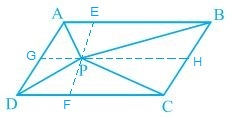Guru

# In Fig. 9.16, P is a point in the interior of a parallelogram ABCD. Show that (i) ar(APB) + ar(PCD) = ½ ar(ABCD) (ii) ar(APD) + ar(PBC) = ar(APB) + ar(PCD) [Hint : Through P, draw a line parallel to AB. Q.4

• 0

How i solve the question of class 9th ncert math of Areas of Parallelograms and Triangles chapter of exercise 9.2of question no 4 . I think it is very important question of class 9th give me the tricky way for solving this question In Fig. 9.16, P is a point in the interior of a parallelogram ABCD. Show that (i) ar(APB) + ar(PCD) = ½ ar(ABCD) (ii) ar(APD) + ar(PBC) = ar(APB) + ar(PCD) [Hint : Through P, draw a line parallel to AB.

Share

1.(i) A line GH is drawn parallel to AB passing through P.

In a parallelogram,

AB || GH (by construction) — (i)

∴,

AD || BC ⇒ AG || BH — (ii)

From equations (i) and (ii),

ABHG is a parallelogram.

Now,

ΔAPB and parallelogram ABHG are lying on the same base AB and in-between the same parallel lines AB and GH.

∴ ar(ΔAPB) = ½ ar(ABHG) — (iii)

also,

ΔPCD and parallelogram CDGH are lying on the same base CD and in-between the same parallel lines CD and GH.

∴ ar(ΔPCD) = ½ ar(CDGH) — (iv)

ar(ΔAPB) + ar(ΔPCD) = ½ [ar(ABHG)+ar(CDGH)]

⇒ ar(APB)+ ar(PCD) = ½ ar(ABCD)

(ii) A line EF is drawn parallel to AD passing through P.

In the parallelogram,

AD || EF (by construction) — (i)

∴,

AB || CD ⇒ AE || DF — (ii)

From equations (i) and (ii),

AEDF is a parallelogram.

Now,

ΔAPD and parallelogram AEFD are lying on the same base AD and in-between the same parallel lines AD and EF.

∴ar(ΔAPD) = ½ ar(AEFD) — (iii)

also,

ΔPBC and parallelogram BCFE are lying on the same base BC and in-between the same parallel lines BC and EF.

∴ar(ΔPBC) = ½ ar(BCFE) — (iv)